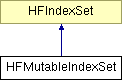# HFMutableIndexSet Class Reference

The mutable subclass of HFIndexSet.

Inheritance diagram for HFMutableIndexSet:List of all members.

## Public Member Functions

(void) - removeIndexesInRange:
(void) - shiftValuesRightByAmount:startingAtValue:
(void) - shiftValuesLeftByAmount:startingAtValue:
(void) - shiftValuesLeftByAmount:endingAtValue:

## Member Function Documentation

Adds indexes in the given range.

 - (void) removeIndexesInRange: (HFRange) range

Removes indexes in the given range.

 - (void) shiftValuesRightByAmount: (unsigned long long) delta startingAtValue: (unsigned long long) value

Shifts all values equal to or greater than the given value right (increase) by the given delta. This raises an exception if indexes are shifted past ULLONG_MAX.

 - (void) shiftValuesLeftByAmount: (unsigned long long) delta startingAtValue: (unsigned long long) value

Shifts all values equal to or greater than the given value left (decrease) by the given delta. Values within the range {value - delta, delta} are deleted. This raises an exception if indexes are shifted below 0.

 - (void) shiftValuesLeftByAmount: (unsigned long long) delta endingAtValue: (unsigned long long) value

Shifts all values less than the given value left (decrease) by the given delta. This raises an exception of indexes are shifted below 0.

The documentation for this class was generated from the following file:

Generated on Thu Dec 8 10:06:35 2011 for HexFiend by1.5.9